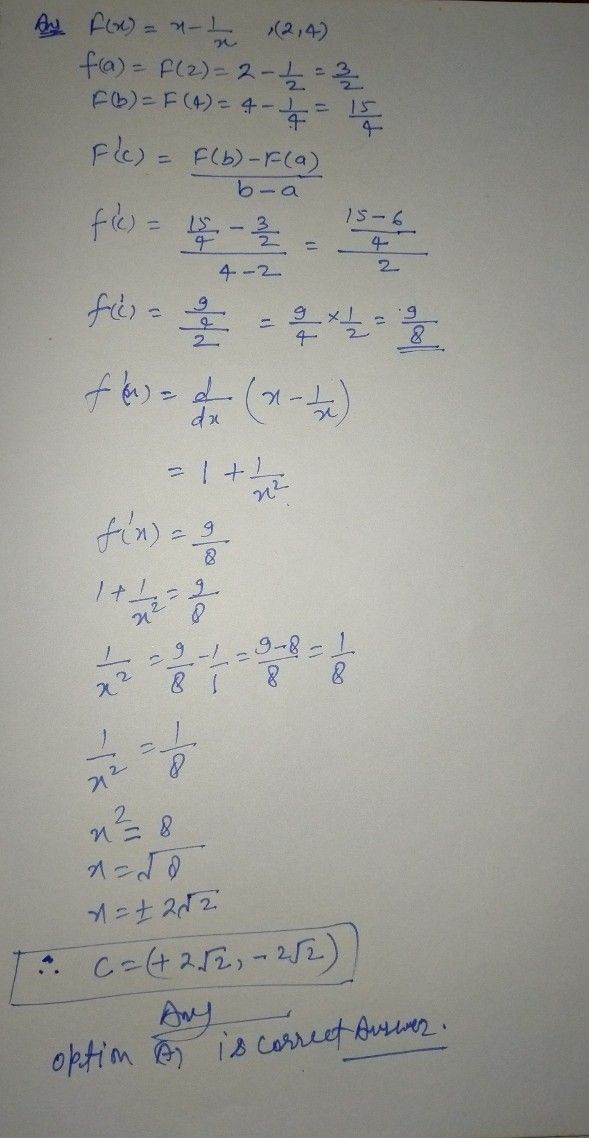Symbol
ProblemWhat is the value of c that satisfies the equation $\dfrac {f\left(b\right)-f\left(a\right)} {b-a}=f^{'}\left(c\right)$ in the conclusion of the Mean Value Theorem for the function $f\left(x\right)=x-\dfrac {1} {x}$ $1$ in the interval $\left(2,4\right)7$ Select $O10P$ O $A.2\sqrt{2}$ $B.9/4$ $C.5/2$ $D.2$ $E.3$
Calculus
SolutionQanda teacher - Balramsingif you understand the question then you are evaluated the question with five star